# 异常值检测！最佳统计方法实践（代码实现）！# 💡 异常值 Q&A## 💦 异常值对分布有什么影响？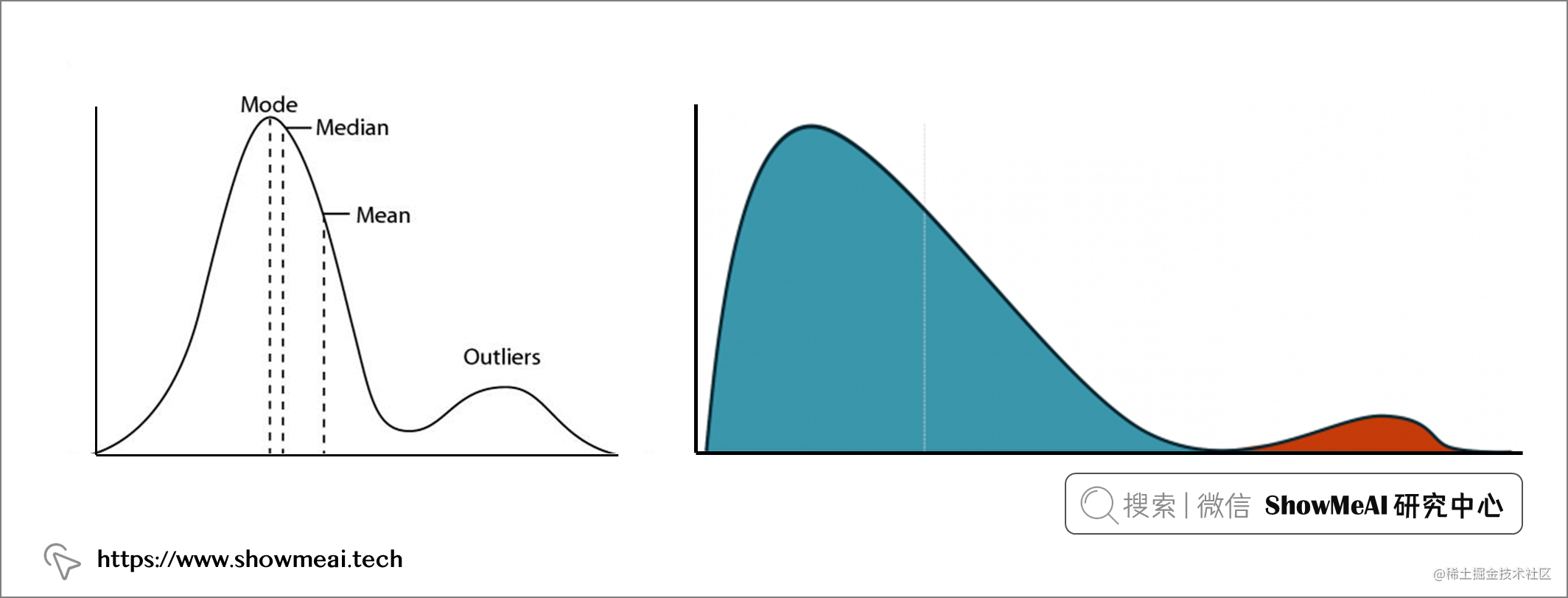## 💦 异常值对机器学习模型有什么影响？

• 如果认为异常值是自然的，不是由于测量错误产生的 → 应该将其保留在数据集中，并用『标准化』等数据预处理方式处理。
• 如果有一个包含少量异常值的大型数据集 → 应该将其保留，不会显著影响结果。
• 如果确定异常值是由测量误差造成的 → 应该将它们从数据集中删除。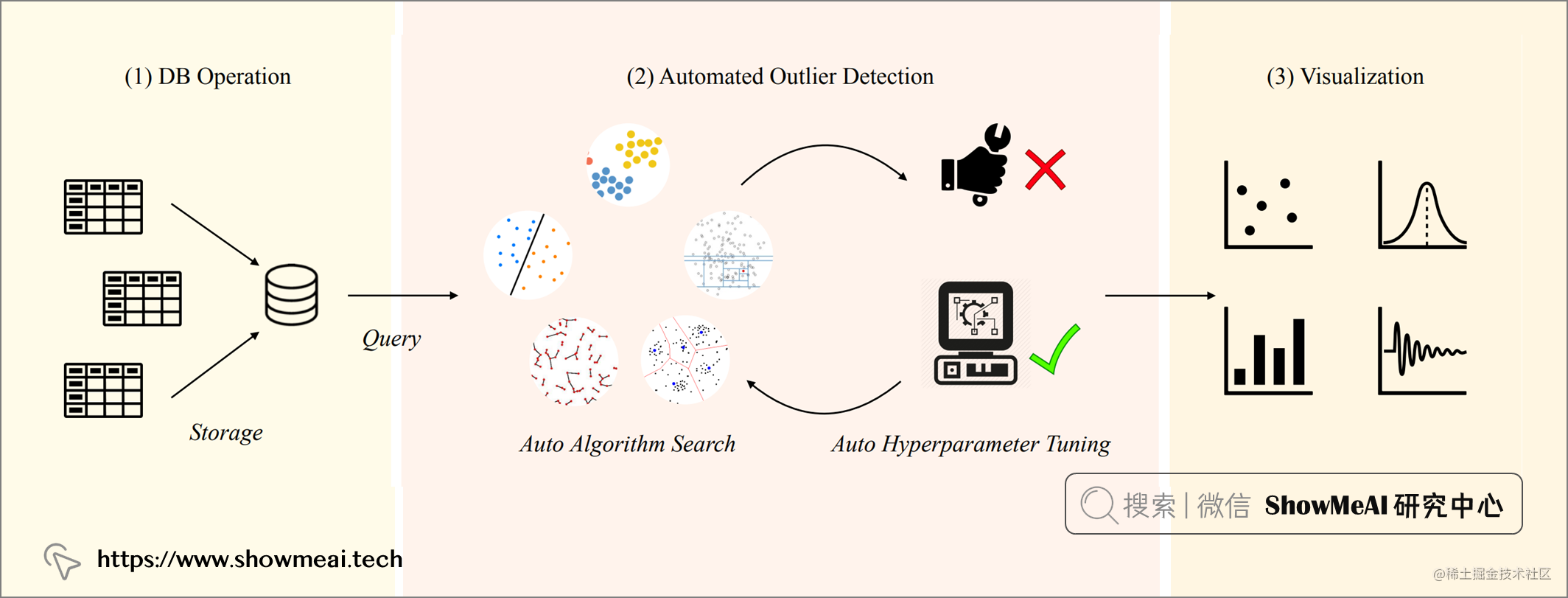# 💡 基于可视化的异常值检测

🏆 实战数据集下载（百度网盘）：公众号『ShowMeAI研究中心』回复『实战』，或者点击 这里 获取本文 基于统计方法的异常值检测代码实战insurance数据集

ShowMeAI官方GitHubgithub.com/ShowMeAI-Hu…

``````import numpy as np
import pandas as pd
import seaborn as sns
df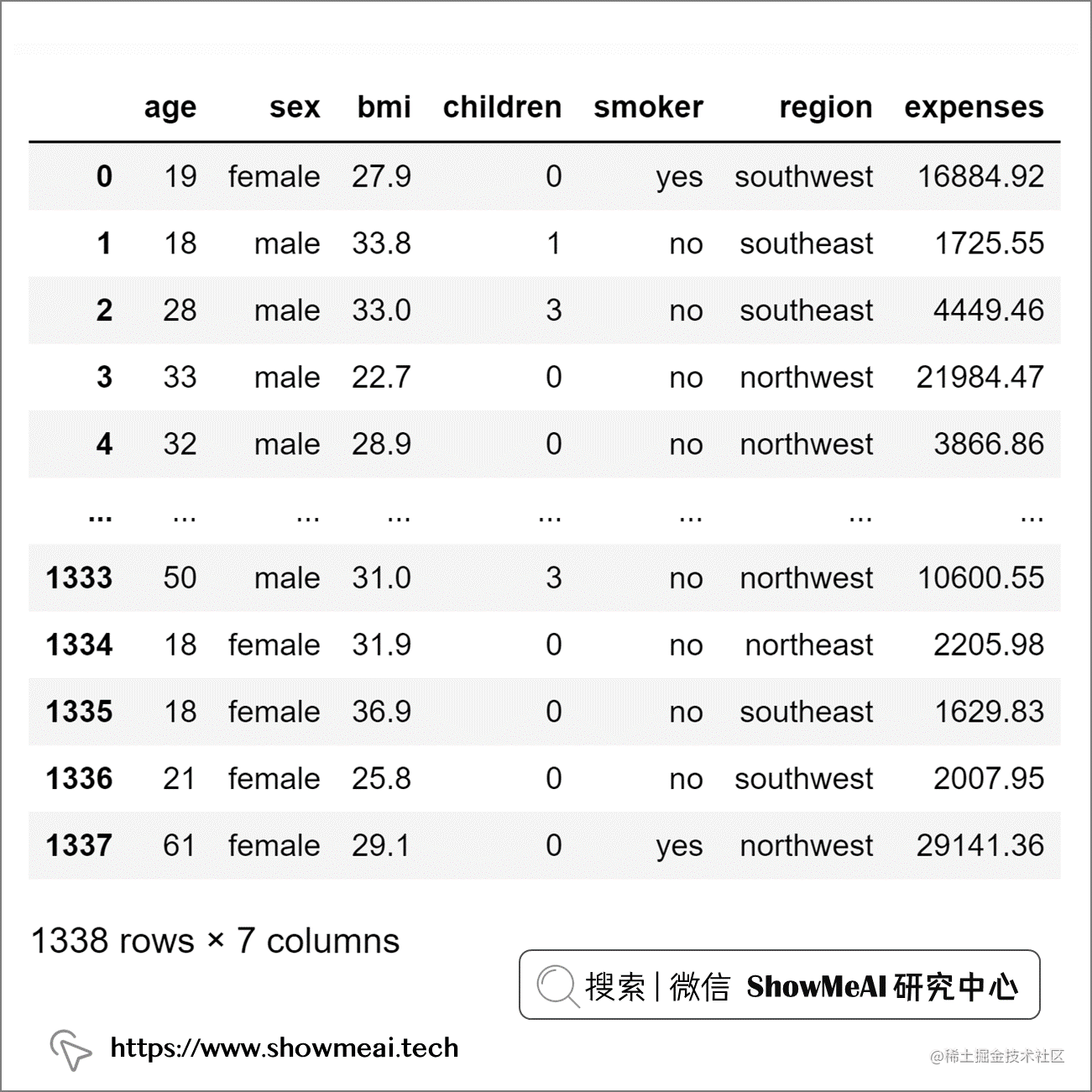``````# age, bmi 和 expenses的箱线图绘图
sns.boxplot(y="age", data=df)
sns.boxplot(y="bmi", data=df)
sns.boxplot(y="expenses", data=df)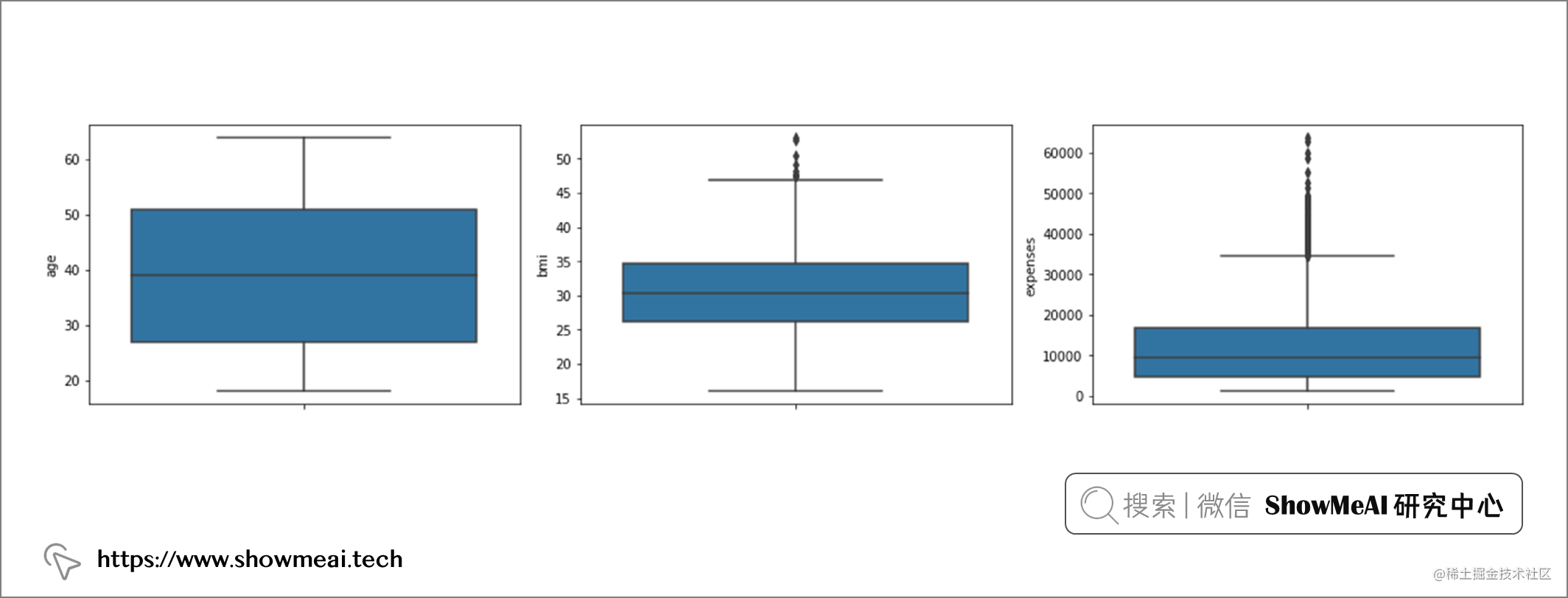``````# age, bmi 和 expenses的直方图
sns.histplot(df, x="age", kde=True)
sns.histplot(df, x="bmi", kde=True)
sns.histplot(df, x="expenses", kde=True)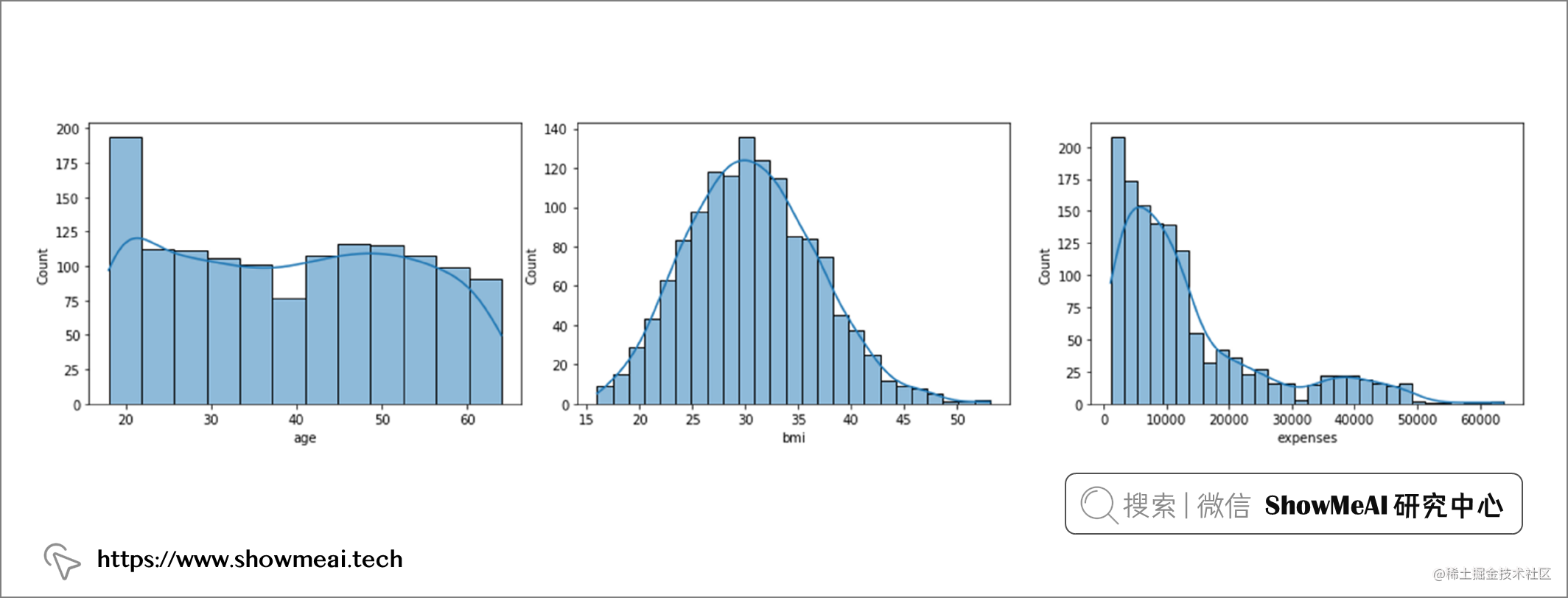``````#bmi 和 expenses 的异常值处理
df.drop(df[df['bmi'] >= 47].index, inplace = True)
df.drop(df[df['expenses'] >= 50000].index, inplace = True)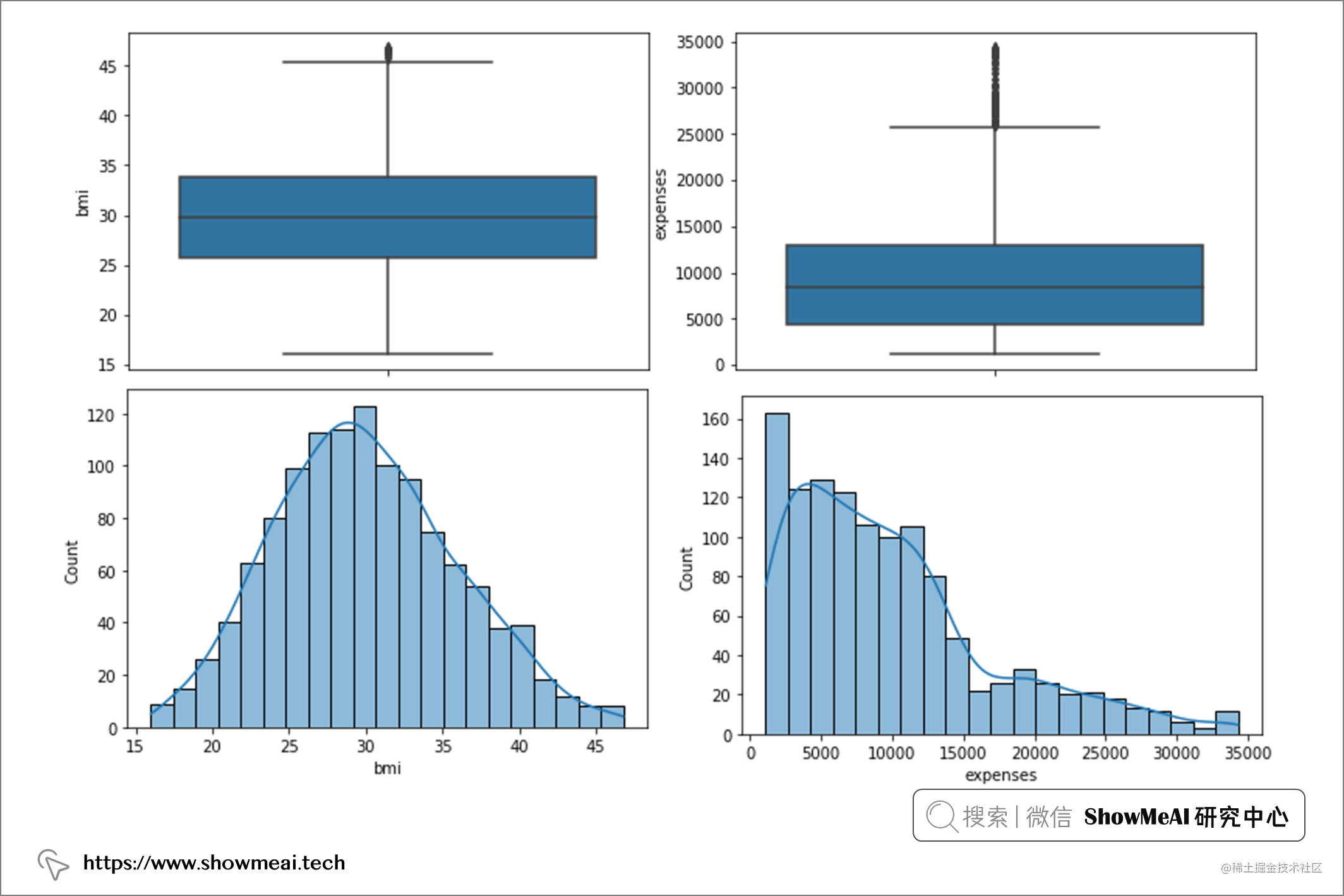# 💡 基于统计方法的异常值检测

## 💦 使用 z 分数检测异常值

Z 分数是一种数学变换，它根据每个观测值与平均值的距离对其进行分类。z-score 的计算公示为：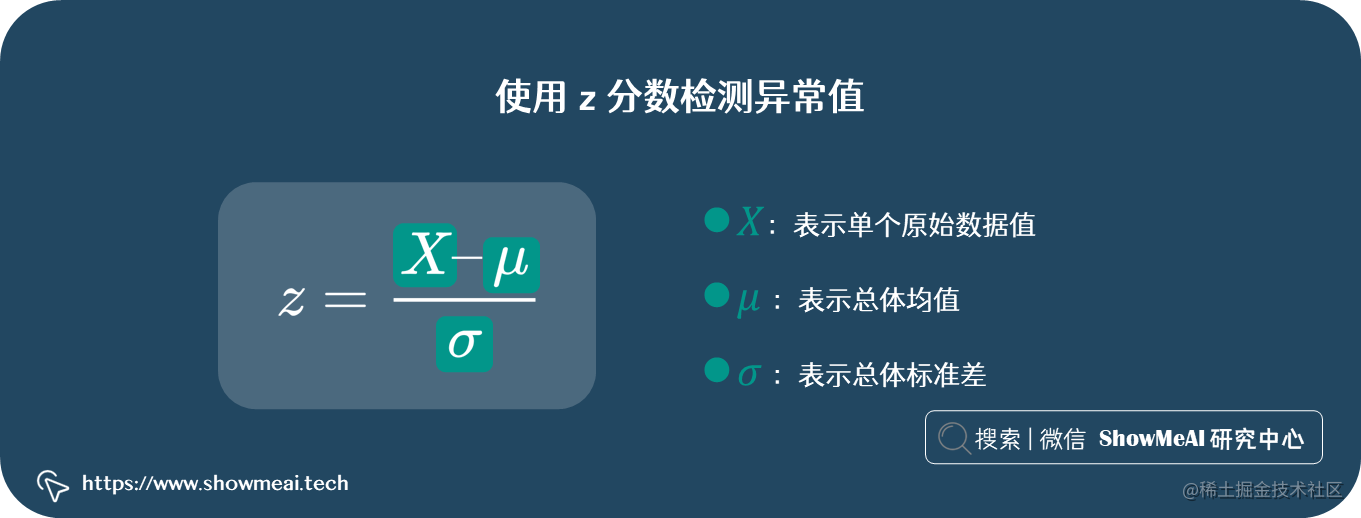``````# 重新加载数据

# 为age计算均值和标准差
mean_age = statistics.mean(df['age'])
stdev_age = statistics.stdev(df['age'])

# 计算z值
age_z_score = (df['age']-mean_age)/stdev_age

# 添加z结果到原dataframe
df['age_z_score'] = age_z_score.tolist()

``````# 检测小于-3SD的值:
df.sort_values(by=['age_z_score'], ascending=True)``````# 检测+3SD以上的值:
df.sort_values(by=['age_z_score'], ascending=False)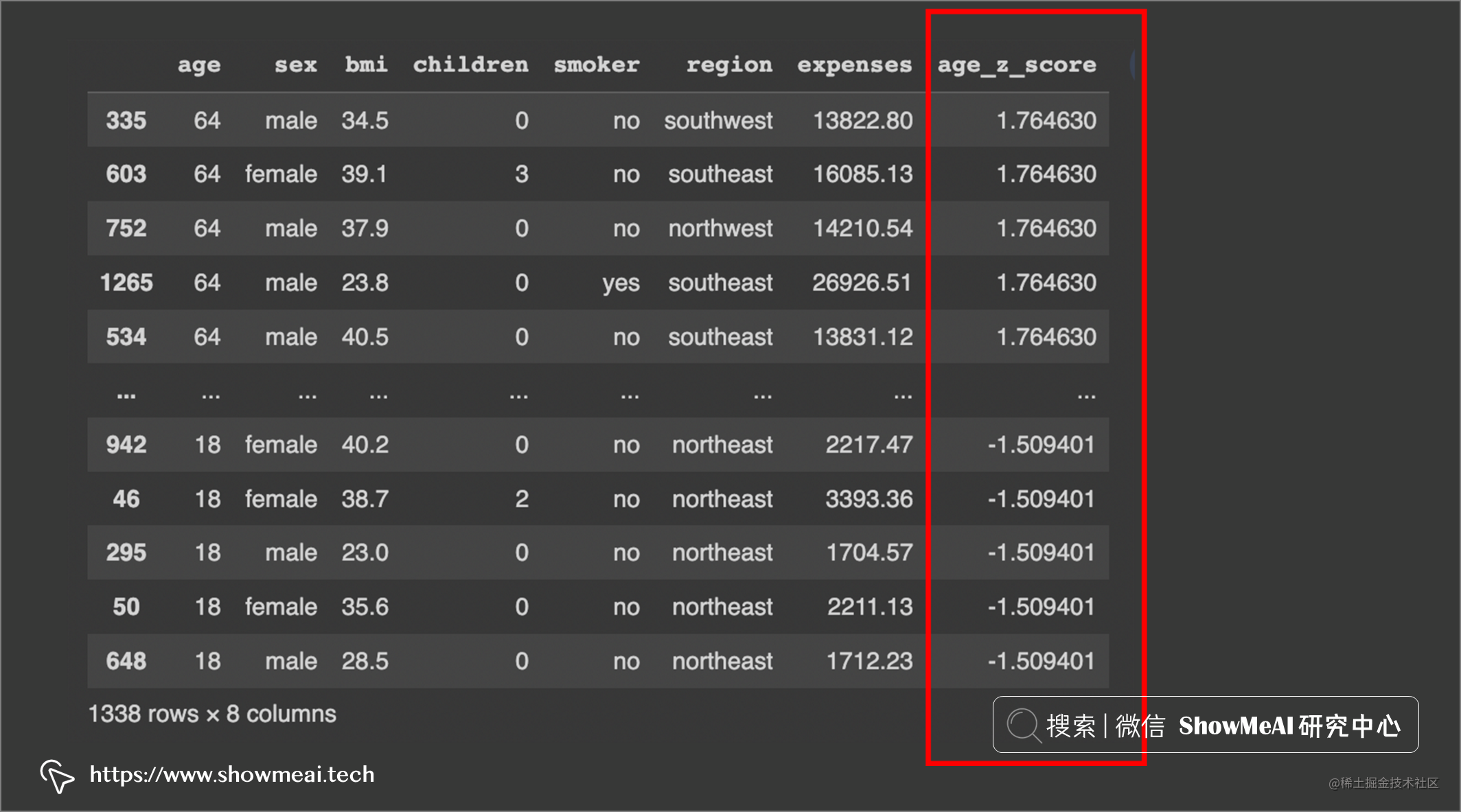``````# 为bmi计算均值和标准差
mean_bmi = statistics.mean(df['bmi'])
stdev_bmi = statistics.stdev(df['bmi'])

# 为bmi计算z-score
bmi_z_score = (df['bmi']-mean_bmi)/stdev_bmi

# 添加到原始dataframe
df['bmi_z_score'] = bmi_z_score.tolist()

# 检查低于-3SD的值
df.sort_values(by=['bmi_z_score'], ascending=True)

# 检查大于3SD的值
df.sort_values(by=['bmi_z_score'], ascending=False)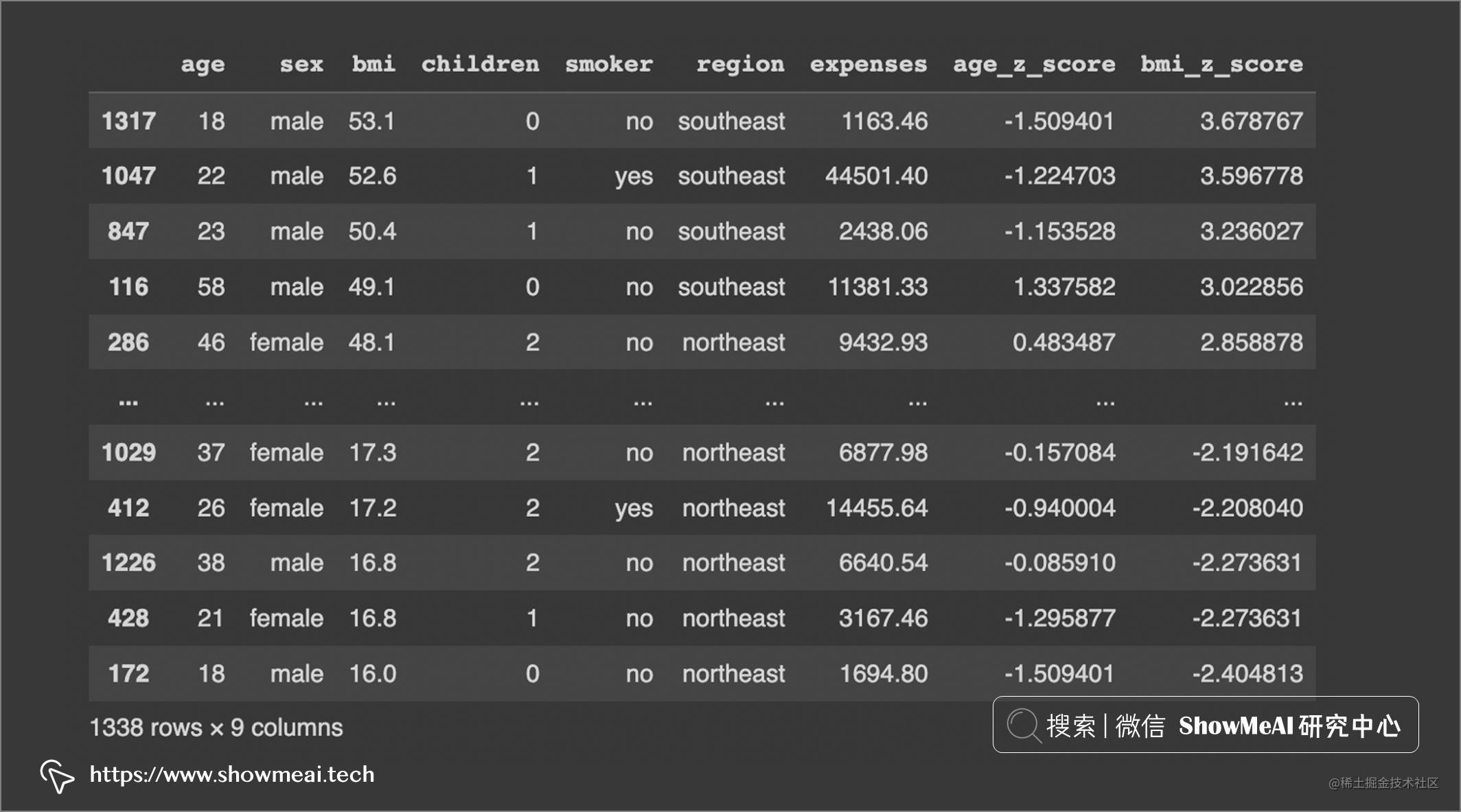``````# 异常值处理
df.drop(df[df[‘bmi_z_score’] >= 3].index, inplace = True)

``````# 为expenses计算均值和标准差
mean_expenses = statistics.mean(df['expenses'])
stdev_expenses = statistics.stdev(df['expenses'])

# 计算z-score
expenses_z_score = (df['expenses']-mean_expenses)/stdev_expenses

# 添加到原始dataframe
df['expenses_z_score'] = expenses_z_score.tolist()

# 检查低于-3SD的值
df.sort_values(by=['expenses_z_score'], ascending=True)

# 检查高于3SD的值
df.sort_values(by=['expenses_z_score'], ascending=False)

# 异常值处理
df.drop(df[df[‘expenses_z_score’] >= 3].index, inplace = True)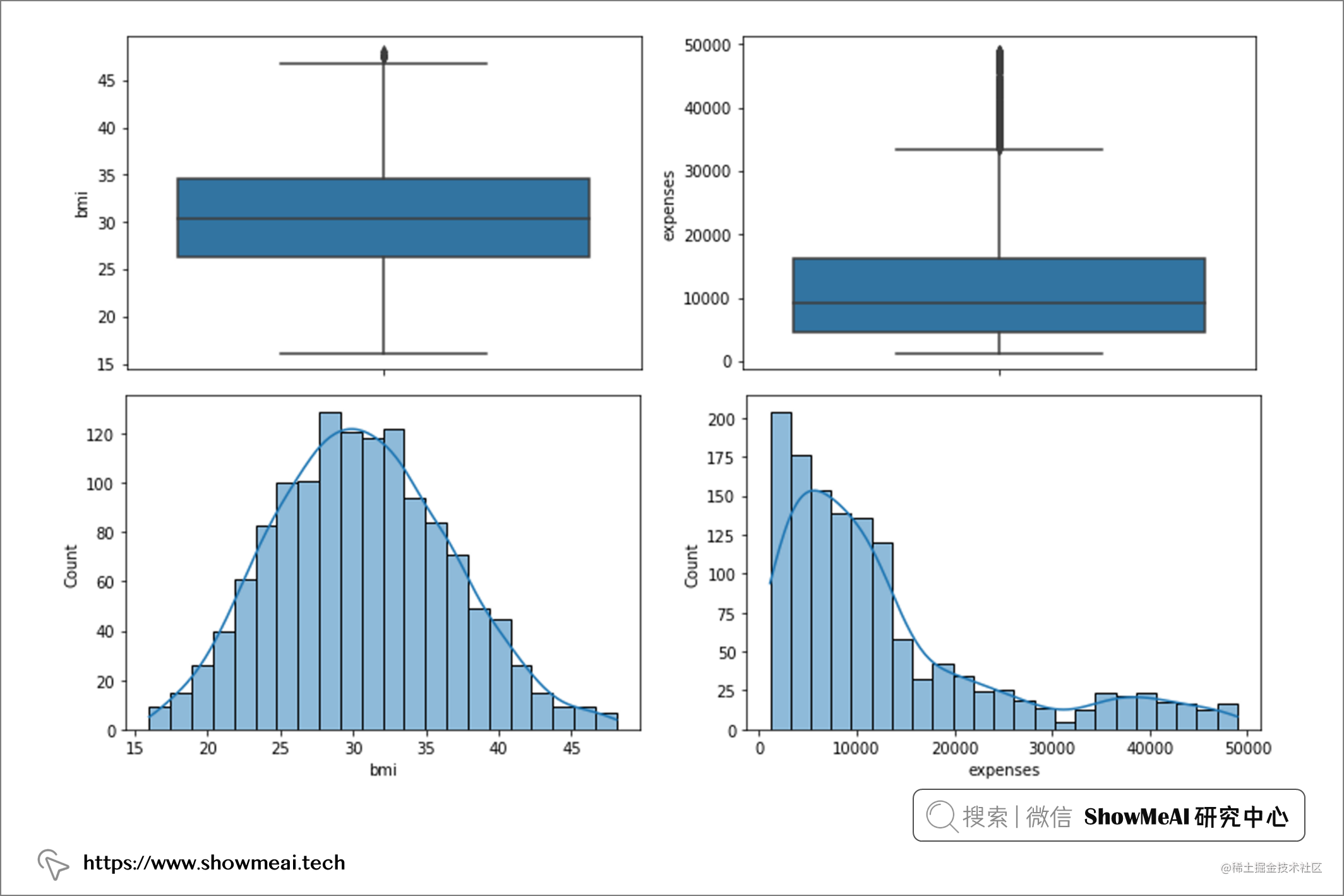## 💦 使用四分位距检测异常值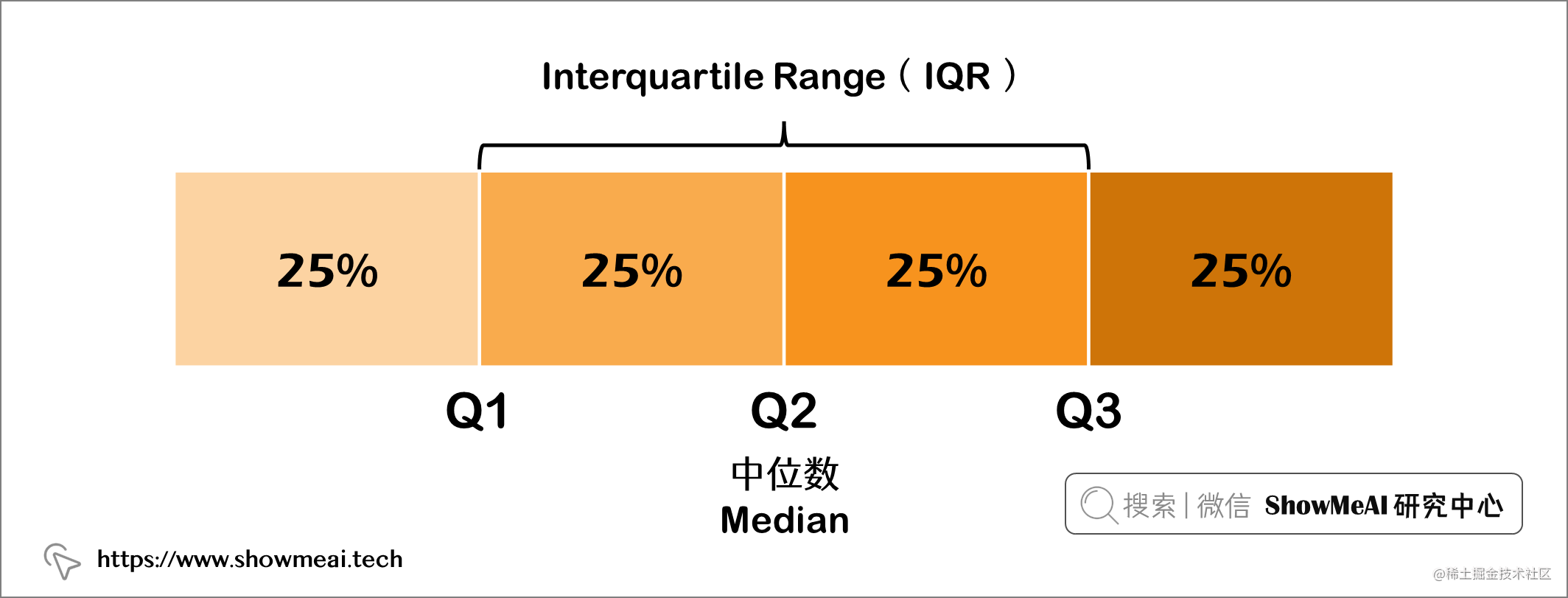``````# 重新加载数据

# 计算上下四分位数位置
q75_age, q25_age = np.percentile(df['age'], [75 ,25])
iqr_age = q75_age - q25_age
iqr_age

# 计算上下边界以用于异常检测
age_h_bound = q75_age+(1.5*iqr_age)
age_l_bound = q25_age-(1.5*iqr_age)
print(age_h_bound)
print(age_l_bound)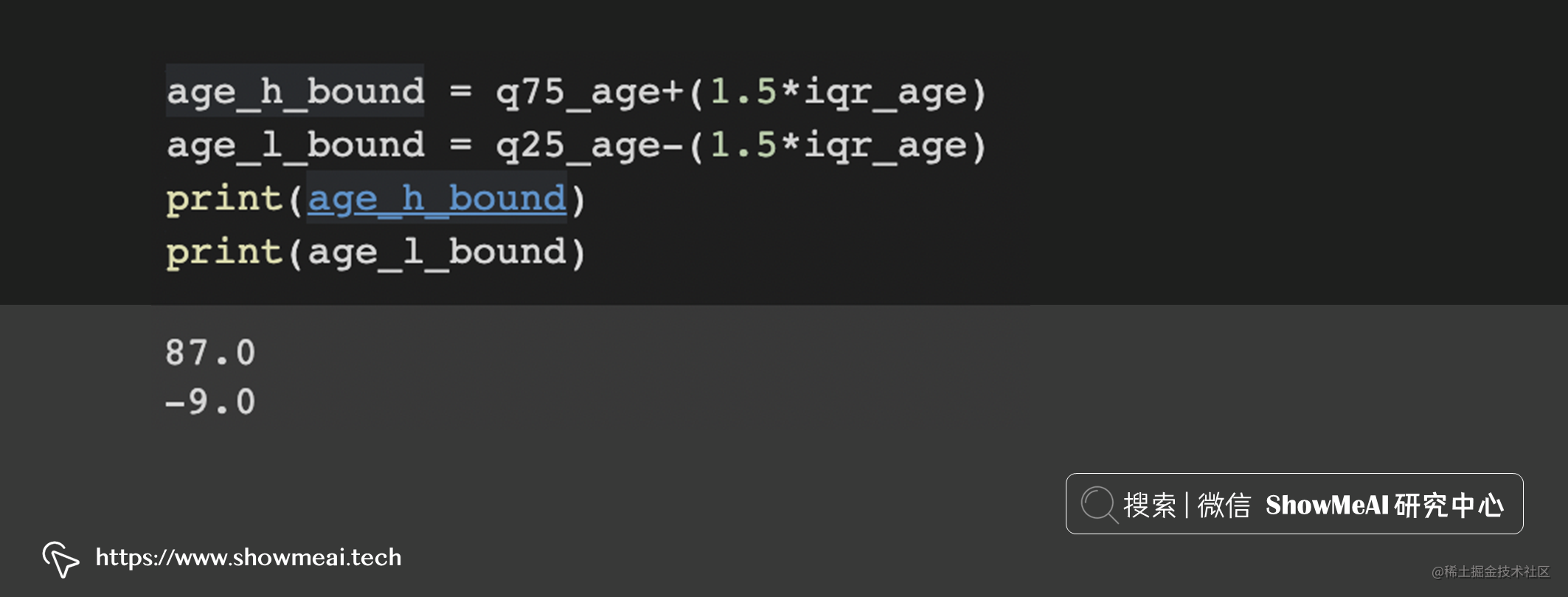``````# 排序
df.sort_values(by=['age'], ascending=True)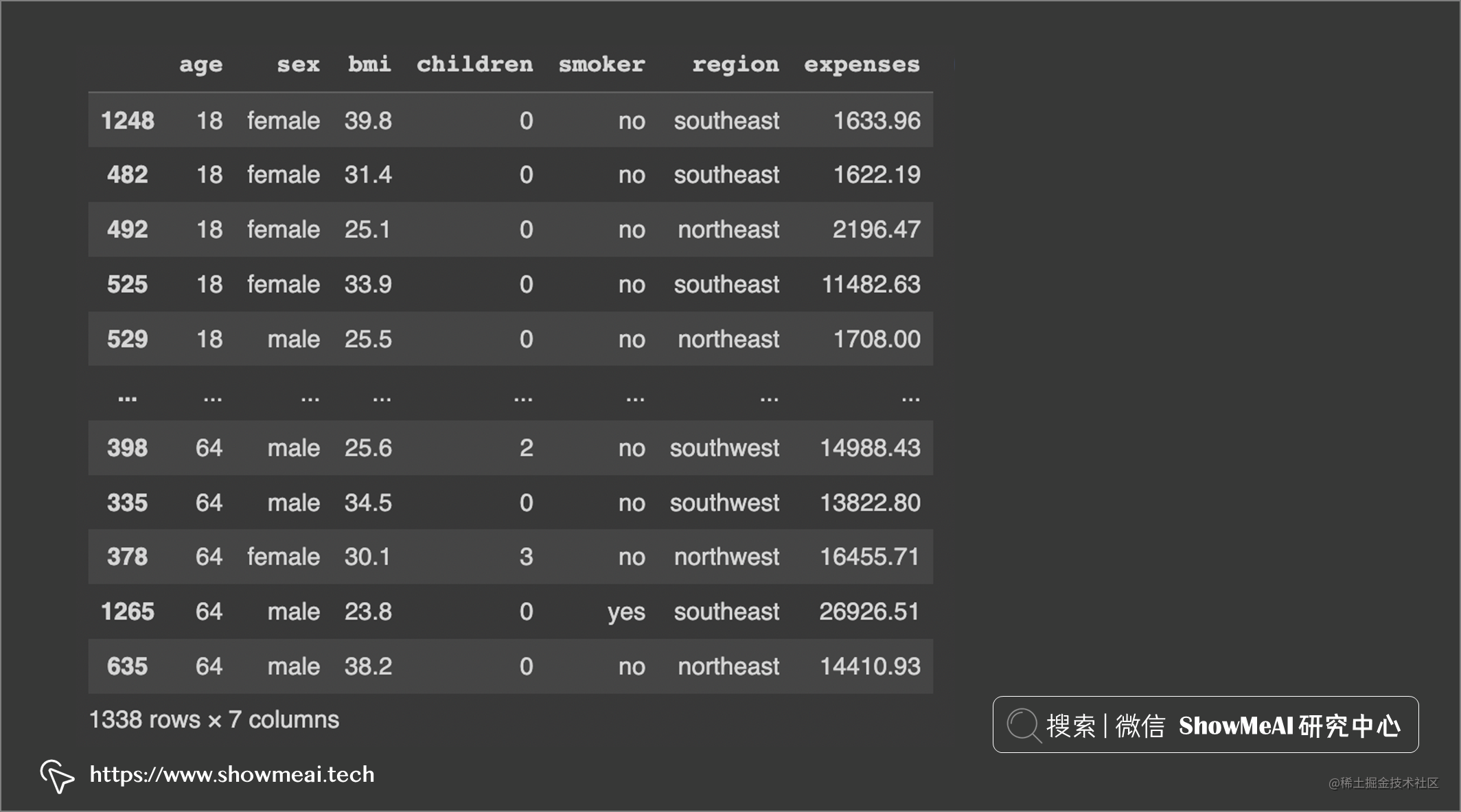``````# 排序
df.sort_values(by=['age'], ascending=False)

``````# 计算上下四分位数位置
q75_bmi, q25_bmi = np.percentile(df['bmi'], [75 ,25])
iqr_bmi = q75_bmi - q25_bmi
iqr_bmi

# 计算上下边界以用于异常检测
bmi_h_bound = q75_bmi+(1.5*iqr_bmi)
bmi_l_bound = q25_bmi-(1.5*iqr_bmi)
print(bmi_h_bound)
print(bmi_l_bound)

# 排序
df.sort_values(by=['bmi'], ascending=True)
df.sort_values(by=['bmi'], ascending=False)

# 剔除异常值
df.drop(df[df['bmi'] >= 47.3].index, inplace = True)
df.drop(df[df['bmi'] <= 13.7].index, inplace = True)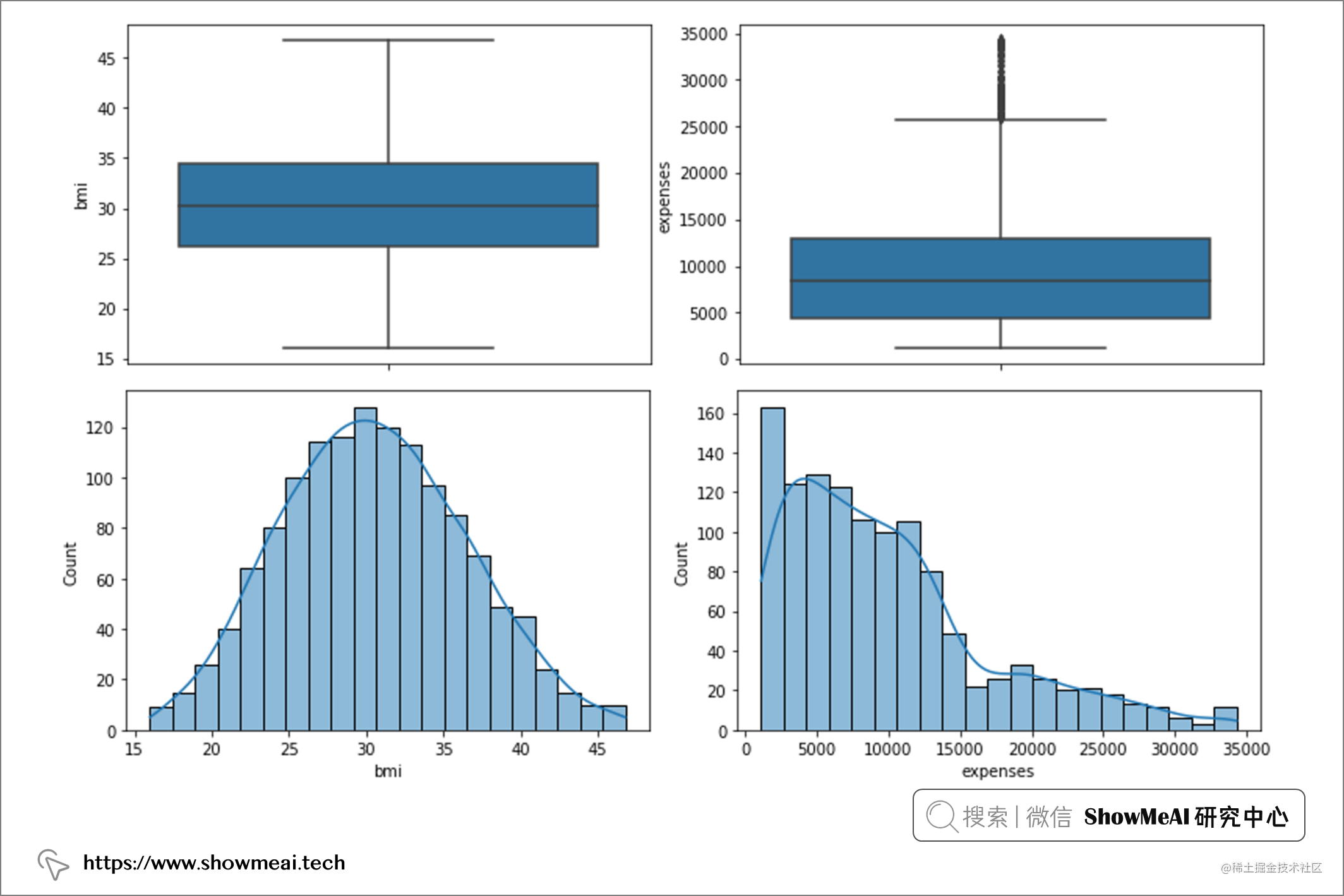# 参考资料